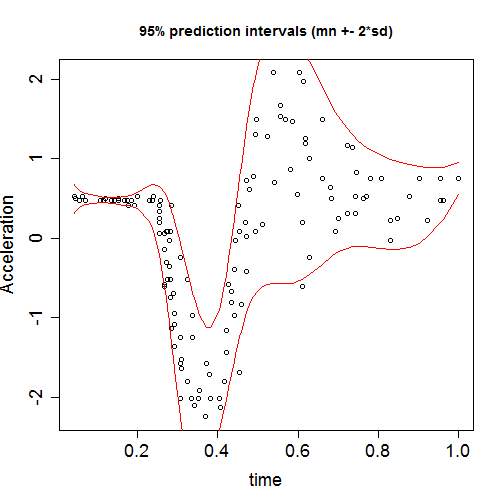# Non-linear regression for the motorcycle data

### Chapter 4.4.1: Nonparametric regression models

In this example $$X$$ is the time since the motorcycle crash and $$Y$$ is the acceleration of the driver's head. We will fit the semiparametric model $Y_{i} \sim \mbox{Normal}[g(X_i),\sigma^2]$ where the mean function $$g$$ is assumed to have spline basis representation $g(X) = \mu + \sum_{j=1}^JB_j(X)\beta_j.$ The remaining parameters have uninformative priors: $$\mu \sim \mbox{Normal}(0,100)$$, $$\beta_j \sim \mbox{Normal}(0,\sigma^2\tau^2)$$ and $$\sigma^2,\tau^2\sim\mbox{InvGamma}(0.1,0.1)$$.

## Load and plot the motorcylce data

 library(MASS)

Y <- mcycle$accel X <- mcycle$times

Y <- (Y-mean(Y))/sd(Y)
X <- X/max(X)

n <- length(Y)
n

##  133

 plot(X,Y,xlab="time",ylab="Acceleration",cex.lab=1.5,cex.axis=1.5)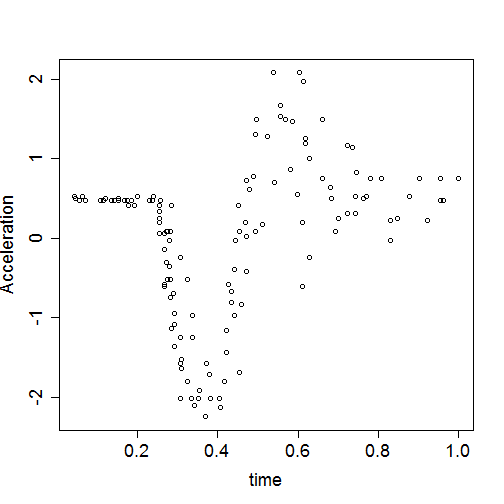## Set up a spline basis expansion

 library(splines)

J <- 10       # Number of basis functions
B <- bs(X,J)  # Specify the basis functions
dim(B)

##  133  10

 matplot(X,B,type="l",
xlab="Time",ylab="Basis function, B_j(X)",
cex.lab=1.5,cex.axis=1.5,lty=1,lwd=2)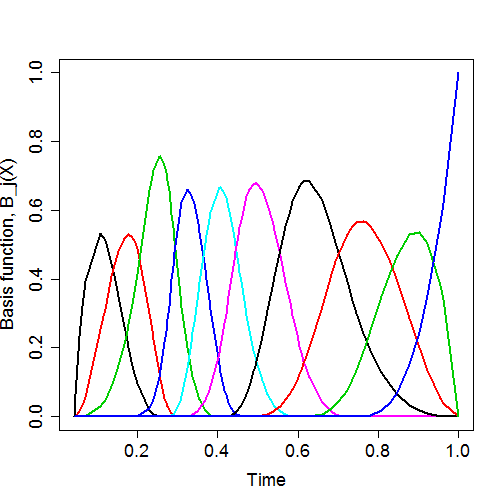Moto_model <- "model{

# Likelihood
for(i in 1:n){
Y[i]    ~ dnorm(mean[i],taue)
mean[i] <- mu + inprod(B[i,],beta[])
}

# Prior
mu   ~ dnorm(0,0.01)
taue ~ dgamma(0.1,0.1)
for(j in 1:J){
beta[j] ~ dnorm(0,taue*taub)
}
taub ~ dgamma(0.1,0.1)

}"


## Fit the model

   library(rjags)
dat    <- list(Y=Y,n=n,B=B,J=J)
init   <- list(mu=mean(Y),beta=rep(0,J),taue=1/var(Y))
model  <- jags.model(textConnection(Moto_model),
inits=init,data = dat,quiet=TRUE)

update(model, 10000, progress.bar="none")

samp   <- coda.samples(model,
variable.names=c("mean"),
n.iter=20000, progress.bar="none")


## Plot the fixed curve, $$g(X)$$

   sum <- summary(samp)
names(sum)

##  "statistics" "quantiles"  "start"      "end"        "thin"
##  "nchain"

   q <- sum$quantiles plot(X,Y,xlab="time",ylab="Acceleration", cex.lab=1.5,cex.axis=1.5) lines(X,q[,1],col=2,lty=2) # 0.025 quantile (lower bound) lines(X,q[,3],col=2,lty=1) # 0.500 quantile (median) lines(X,q[,5],col=2,lty=2) # 0.975 quantile (upper bound) legend("bottomright",c("Median","95% interval"), lty=1:2,col=2,bg=gray(1),inset=0.05,cex=1.5)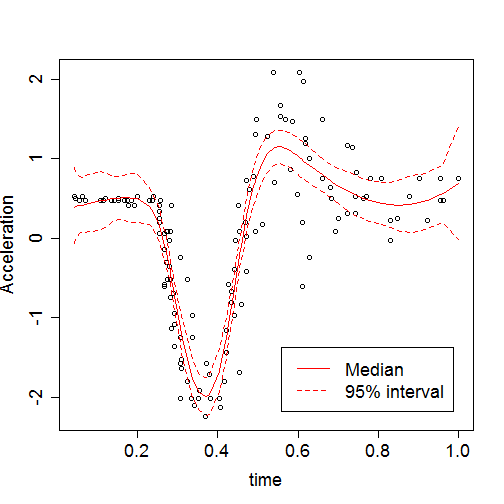Summary: The mean trend seems to fit the data well. However, the variance of the observations around the mean varies with $$X$$. ## Heteroskedastic model The variance is small for $$X$$ near zero and increases with $$X$$. To account for this, we allow the log of the variance to vary with $$X$$ following a second spline basis expansion: $Y_{i} \sim \mbox{Normal}[g(X_i),\sigma^2(X_i)]$ where $$g(X) = \mu + \sum_{j=1}^JB_j(X)\beta_j$$ is modelled as above and $log[\sigma^2(X)] = \mu_2 + \sum_{j=1}^JB_j(X)\alpha_j.$ The parameters have uninformative priors $$\mu_k \sim \mbox{Normal}(0,100)$$, $$\beta_j \sim \mbox{Normal}(0,\sigma_b^2)$$, $$\alpha_j \sim \mbox{Normal}(0,\sigma_a^2)$$ and $$\sigma_a^2,\sigma_b^2\sim\mbox{InvGamma}(0.1,0.1)$$. Moto_model2 <- "model{ # Likelihood for(i in 1:n){ Y[i] ~ dnorm(mean[i],inv_var[i]) mean[i] <- mu1 + inprod(B[i,],beta[]) inv_var[i] <- 1/sig2[i] log(sig2[i]) <- mu2 + inprod(B[i,],alpha[]) } # Prior mu1 ~ dnorm(0,0.01) mu2 ~ dnorm(0,0.01) for(j in 1:J){ beta[j] ~ dnorm(0,taub) alpha[j] ~ dnorm(0,taua) } taua ~ dgamma(0.1,0.1) taub ~ dgamma(0.1,0.1) # Prediction intervals for(i in 1:n){ low[i] <- mean[i] - 1.96*sqrt(sig2[i]) high[i] <- mean[i] + 1.96*sqrt(sig2[i]) } }"  ## Fit the model  library(rjags) dat <- list(Y=Y,n=n,B=B,J=J) init <- list(mu1=mean(Y),beta=rep(0,J), mu2=log(var(Y)),alpha=rep(0,J)) model <- jags.model(textConnection(Moto_model2), inits=init,data = dat, quiet=TRUE) update(model, 10000, progress.bar="none") samp2 <- coda.samples(model, variable.names=c("mean","sig2","low","high"), n.iter=20000, progress.bar="none")  ## Plot the fixed curve, $$g(X)$$  q2 <- summary(samp2)$quantiles
high <- q2[1:n+0*n,]
low  <- q2[1:n+1*n,]
mean <- q2[1:n+2*n,]
sig2 <- q2[1:n+3*n,]

plot(X,Y,xlab="time",ylab="Acceleration",
main="Fitted mean trend",
cex.lab=1.5,cex.axis=1.5)

lines(X,mean[,1],col=2,lty=2) # 0.025 quantile (lower bound)
lines(X,mean[,3],col=2,lty=1) # 0.500 quantile (median)
lines(X,mean[,5],col=2,lty=2) # 0.975 quantile (upper bound)

legend("bottomright",c("Median","95% interval"),
lty=1:2,col=2,bg=gray(1),inset=0.05,cex=1.5)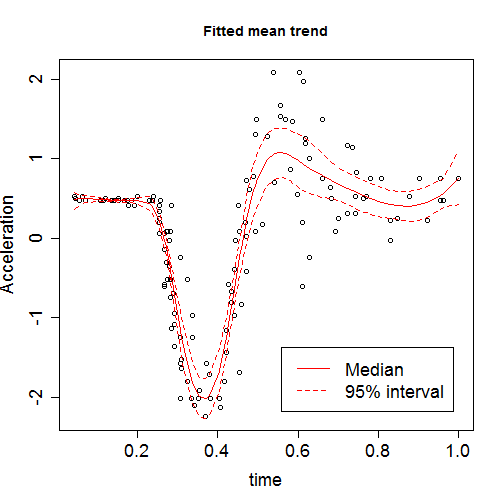plot(NA,xlim=c(0,1),ylim=c(0,2),
xlab="time",ylab="Variance",
main="Fitted variance",
cex.lab=1.5,cex.axis=1.5)

lines(X,sig2[,1],col=2,lty=2) # 0.025 quantile (lower bound)
lines(X,sig2[,3],col=2,lty=1) # 0.500 quantile (median)
lines(X,sig2[,5],col=2,lty=2) # 0.975 quantile (upper bound)

legend("topleft",c("Median","95% interval"),
lty=1:2,col=2,bg=gray(1),inset=0.05,cex=1.5)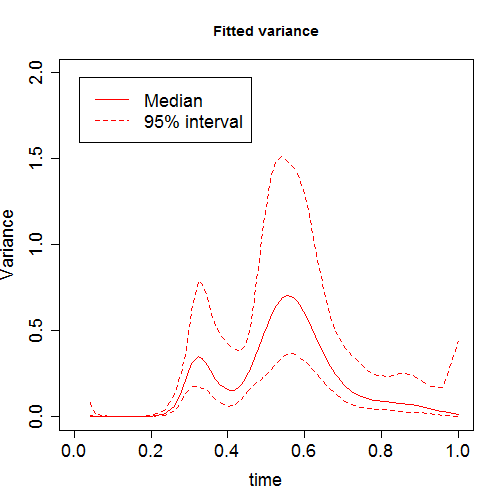plot(X,Y,xlab="time",ylab="Acceleration",
main="95% prediction intervals (mn +- 2*sd)",
cex.lab=1.5,cex.axis=1.5)

lines(X,low[,3],col=2,lty=1) # 0.500 quantile (median)
lines(X,high[,3],col=2,lty=1) # 0.500 quantile (median)